# Theoretical Plate Number and Symmetry Factor

"Theoretical plate number" and "symmetry factor" are important indexes that describe column performance. This article talks about these two indexes.

## Theoretical Plate Number

Theoretical plate number (N) is an index that indicates column efficiency. It describes the number of plates as defined according to plate theory, and can be used to determine column efficiency based on calculation in which the larger the theoretical plate number the sharper the peaks. The theoretical plate number is included as a numerical value in column instruction manuals and inspection reports. Assuming a Gaussian distribution (normal distribution), the theoretical plate number is represented by equation (1).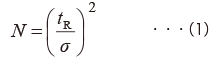Where tR is retention time and σ is standard deviation. As shown in Fig. 1, assuming a Gaussian distribution, the peak width W is 4 σ and peak FWHM W0.5h is 2.354 σ. Substituting these values into equation (1) gives equations (2) and (3).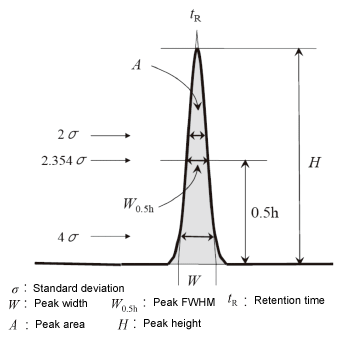Fig. 1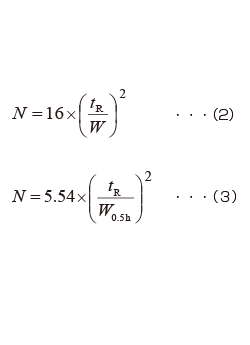The theoretical plate number can also be represented in terms of peak area A and peak heightHas shown in equation (4).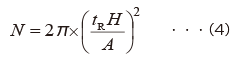## Symmetry Factor

Symmetry factor (S, also called "tailing factor") is a coefficient that shows the degree of peak symmetry. It is represented in equation (5) based on the measurements shown in Fig. 2.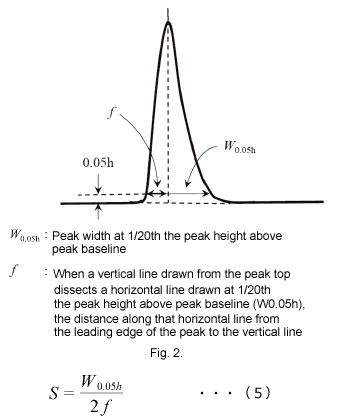S>1: Tailing peak
S=1: Peak with Gaussian distribution (symmetry)